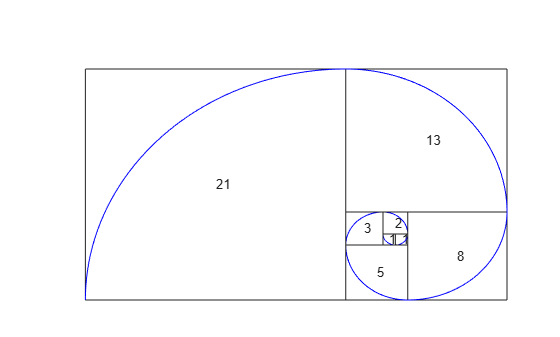fibonacci

Fibonacci numbers

Description

example

fibonacci(n) returns the nth Fibonacci Number.

Examples

Find Fibonacci Numbers

Find the sixth Fibonacci number by using fibonacci.

fibonacci(6)
ans =
8

Find the first 10 Fibonacci numbers.

n = 1:10;
fibonacci(n)
ans =
1     1     2     3     5     8    13    21    34    55

Fibonacci Sequence Approximates Golden Ratio

The ratio of successive Fibonacci numbers converges to the golden ratio $1.61803...$. Show this convergence by plotting this ratio against the golden ratio for the first 10 Fibonacci numbers.

n = 2:10;
ratio = fibonacci(n)./fibonacci(n-1);

plot(n,ratio,'--o')
hold on

line(xlim,[1.618 1.618])
hold offSymbolically Represent Fibonacci Numbers

Use Fibonacci numbers in symbolic calculations by representing them with symbolic input. fibonacci returns the input.

Represent the nth Fibonacci number.

syms n
fibonacci(n)
ans =
fibonacci(n)

Find Large Fibonacci Numbers

Find large Fibonacci numbers by specifying the input symbolically using sym. Symbolic input returns exact symbolic output instead of double output. Convert symbolic numbers to double by using the double function.

Find the 300th Fibonacci number.

num = sym(300);
fib300 = fibonacci(num)
fib300 =
222232244629420445529739893461909967206666939096499764990979600

Convert fib300 to double. The result is a floating-point approximation.

double(fib300)
ans =
2.2223e+62

For more information on symbolic and double arithmetic, see Choose Numeric or Symbolic Arithmetic.

Golden Spiral Using Fibonacci Numbers

The Fibonacci numbers are commonly visualized by plotting the Fibonacci spiral. The Fibonacci spiral approximates the golden spiral.

Approximate the golden spiral for the first 8 Fibonacci numbers. Define the four cases for the right, top, left, and bottom squares in the plot by using a switch statement. Form the spiral by defining the equations of arcs through the squares in eqnArc. Draw the squares and arcs by using rectangle and fimplicit respectively.

x = 0;
y = 1;
syms v u

axis off
hold on

for n = 1:8

a = fibonacci(n);

% Define squares and arcs
switch mod(n,4)
case 0
y = y - fibonacci(n-2);
x = x - a;
eqnArc = (u-(x+a))^2 + (v-y)^2 == a^2;
case 1
y = y - a;
eqnArc = (u-(x+a))^2 + (v-(y+a))^2 == a^2;
case 2
x = x + fibonacci(n-1);
eqnArc = (u-x)^2 + (v-(y+a))^2 == a^2;
case 3
x = x - fibonacci(n-2);
y = y + fibonacci(n-1);
eqnArc = (u-x)^2 + (v-y)^2 == a^2;
end

% Draw square
pos = [x y a a];
rectangle('Position', pos)

xText = (x+x+a)/2;
yText = (y+y+a)/2;
text(xText, yText, num2str(a))

% Draw arc
interval = [x x+a y y+a];
fimplicit(eqnArc, interval, 'b')

endInput Arguments

collapse all

Input, specified as a number, vector, matrix or multidimensional array, or a symbolic number, variable, vector, matrix, multidimensional array, function, or expression.

collapse all

Fibonacci Number

The Fibonacci numbers are the sequence 0, 1, 1, 2, 3, 5, 8, 13, 21….

Given that the first two numbers are 0 and 1, the nth Fibonacci number is

Fn = Fn–1 + Fn–2.

Applying this formula repeatedly generates the Fibonacci numbers.

Introduced in R2017a

Symbolic Math Toolbox Documentation

Mathematical Modeling with Symbolic Math Toolbox

Get examples and videos